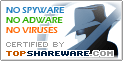# MathX 2.0

SNAPSHOT

Math grapher

Windows AllPlatform :
\$0Price :
827 KBFile Size :
ScreenshotScreenshot :Popularity :Well.. a little program very usefull for students.
It easyly create 2D or 3D graphic of the mathematical functions !

This calculator calculates definite integrals
• TQ's FunPlot
It is a function plotting software that plots any user-typed 1-D and 2-D functions. The generated plots are fully-interactive and document-ready.
• smartCalc
orangeCalc 2005 small calculator
• Visual Complex
Visual Complex is a graph software to create graph of complex function.
• Grab It
Digitize data from graphs and charts or measure distances and angles on pictures
• Math Stars Plus
Practice math facts, other math games
• vCalc
RPN scientific calculator with keyboard UI
• Prime Number Spiral
For exploring the Prime Number Spiral.
• Integrals Ebook
integrals : learn how to solve it
• Guzinta Math
Free Windows and Macintosh desktop lesson apps.